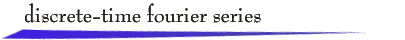Introduction

A real, N-periodic, discrete-time signal x[n] can be represented by a linear combination of the complex exponential signals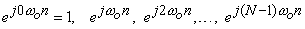as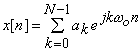In these expressions,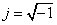, and the discrete-time fundamental frequency is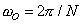. This discrete-time Fourier series representation provides notions of frequency content of discrete-time signals, and it is very convenient for calculations involving linear, time-invariant systems because complex exponentials are eigenfunctions of LTI systems.

The complex coefficients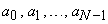can be calculated from the expression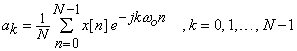The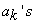are called the spectral coefficients of the signal x[n].  A plot of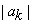vs k is called the magnitude spectrum of x[n], and a plot of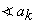vs k is called the phase spectrum of x[n]. These plots, particularly the magnitude spectrum, provide a picture of the frequency composition of the signal. Notice that the spectral coefficients repeat as k is varied. In particular, for any value of k,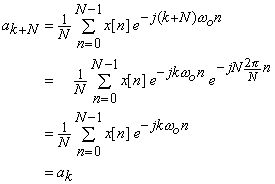First Applet - Entering Signals

First Applet - Entering Spectra

This applet illustrates the discrete-time Fourier series representation for N = 5. Display windows show

·        two repetitions of the magnitude and phase spectra,

·        the individual frequency components (often called phasors)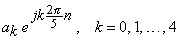·        the sum of these phasor components,

·        two periods of the signal x[n].

You can enter the magnitude and phase spectra with the mouse, and then observe the phasor frequency components and the generation of the signal from these frequency components.  Or you can enter a signal x[n] with the mouse and observe the corresponding frequency spectra and the generation of the signal from the phasor frequency components.

Exercises

(1) Repetition of the spectral coefficients as shown above implies that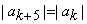. What other patterns or symmetries do you observe in the magnitude spectrum? Can you justify your answer mathematically?

(2) Repetition of the spectral coefficients implies that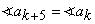. What other patterns or symmetries do you observe in the phase spectrum? Can you justify your answer mathematically?

(3) Using (1) and (2), explain why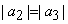and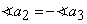for every (real) signal x[n].

(4) Suppose x[n] is even, that is, x[-n] = x[n]. What can you conclude about the spectral coefficients? Can you justify your answer mathematically? (For convenience of signal entry, it is convenient to express the even property as x[n] = x[5-n].)

(5) Suppose x[n] has exactly one nonzero value per period. What do you observe about the magnitude spectrum? Does it matter where the nonzero value occurs? Can you justify your answer mathematically?

(6) If x[n] has exactly one nonzero value per period, what do you observe about the phase spectrum? Does it matter where the nonzero value occurs?

Second Applet

For this applet, you can enter a value of N between 4 and 32, and then enter either a signal or the frequency spectra. Only one period of the signal and one repetition of the spectra are shown.

Exercises

(1) If the period N is an even integer and x[n+N/2] = - x[n], what pattern do you observe in the magnitude spectrum? Can you justify your answer mathematically?

(2) If the N is an even integer and x[n+N/2] =  x[n], what pattern do you observe in the magnitude spectrum? Can you justify your answer mathematically?

(3) If N = 20, what frequencies correspond to the spectral coefficients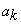for k = 0, 9, and 19? Which of these frequencies would you call “high” frequencies and which would you call “low?”

(4) If N = 20, what is the signal that has all spectral coefficients zero except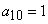? What is the signal if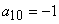?

(5) If N is divisible by 4, what are the spectral coefficients corresponding to sine waves with periods of N, N/2, N/4?return to demonstrations page

 Applets by Lan Ma.